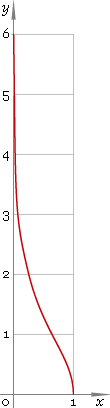The Art of Interface

# arsech or arsch — arc-hyperbolic secant function

Category. Mathematics.

Abstract. Arc-hyperbolic secant: definition, graph, properties and identities.

## 1. Definition

Arc-hyperbolic secant is inverse of hyperbolic secant function. With the help of natural logarithm it can be represented as:

arsechx ≡ ln{[1 + √(1 − x2)] /x}

## 2. Graph

Arc-hyperbolic secant is monotone function defined in the range (0, 1], point x = 0 is singular one. Its graph is depicted below — fig. 1.Fig. 1. Graph of the arc-hyperbolic secant function y = arsechx.

Function codomain is non-negative part of real axis: [0, +∞).

## 3. Identities

Reciprocal argument:

arsech(1/x) = arcoshx

Sum and difference:

arsechx + arsechy = arsech(xy /{1 + √[(1 − x2)(1 − y2)]})
arsechx − arsechy = arsech(xy /{1 − √[(1 − x2)(1 − y2)]})

## 4. Support

Arc-hyperbolic secant function arsech or arsch is supported in:

Arc-hyperbolic secant function of the complex argument arsech or arsch is supported in:

## 5. How to use

To calculate arc-hyperbolic secant of the number:

``arsech(1);``

To calculate arc-hyperbolic secant of the current result:

``arsech(Rslt);``

To calculate arc-hyperbolic secant of the number x in memory:

``arsech(Mem[x]);``